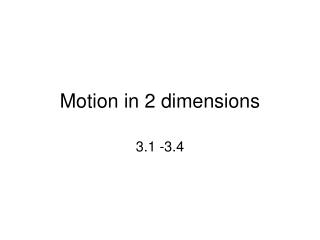# Motion in 2 dimensions - PowerPoint PPT PresentationDownload PresentationMotion in 2 dimensions

Motion in 2 dimensions
Download Presentation## Motion in 2 dimensions

- - - - - - - - - - - - - - - - - - - - - - - - - - - E N D - - - - - - - - - - - - - - - - - - - - - - - - - - -
##### Presentation Transcript

1. Motion in 2 dimensions 3.1 -3.4

2. Vectors vs. Scalars • Scalar- a quantity described by magnitude only. • Given by numbers and units only. • Ex. Distance, speed and mass • Vector – a quantity described by both magnitude and direction • Numbers, units and direction (either words or angles • Ex. Displacement, velocity, acceleration and force

3. vectors • Drawn with an arrow • Length indicates magnitude • Direction pointed • Written in text either • As a boldfaced letter • Or as a letter with an arrow on top. 35 m East

4. Vector subtraction Scalar multiplication • Vectors may be subtracted by adding the opposite (or negative) of the second vector. V2– V1 = V2 + (– V1) • This means that V1 is facing the opposite direction it was originally heading • Multiplying a vector by a scalar simply magnifies its length if V= 3 m then 3V = 9 m

5. Vector addition • Vectors may be added two ways: • One by graphing • Vectors are drawn so that they remain in the same orientation but are placed tip to tail. • Or by the parallelogram method • One by adding the components of each and doing the Pythagorean theorem. • The resultant vector is the outcome of adding 2 vectors together.

6. Parallelogram method: • In the parallelogram method for vector addition, the vectors are translated, (i.e., moved) to a common origin and the parallelogram constructed as follows: • The resultant R is the diagonal of the parallelogram drawn from the common origin.

7. R = resultant vector Pythagorean theorem 5 2 + 10 2 = R 2 R = 11.2 Km Θ = tan -1 (5/10) =26.6° west of north So sum = 11.2 Km @ 26.6° west of north

8. Component method – make a right triangle out of the individual vector

9. The tip of the x-component vector is directly below the tip of the original vector. components

10. . components • ,

11. When adding 2 vectors by the component method • Find the x and y components of each vector • Organize in a table • Then add x component to x component and y component to y component,

12. Use negative x components when vector is pointed to the left • Use Negative y components pointed down • Use the Pythagorean formula to find the length of the resulting vector. • Find the angle using the Tan-1 (Yr /Xr)

13. vector addition applet • simulator

14. What is the vertical component of a 33 m vector that is at a 76° angle with the x axis? y –comp y = sin 76 33 y = 33 sin 76 = 32 m Example 1 33 m 76°

15. A plane is heading to a destination 1750 due north at 175 km/hr in a westward wind blowing 25 km/hr. At what angle should the plane be oriented so that it reaches its destination? The wind will push the plane off course to the west by an angle of tan-1 (25/175) = 8° west of north so the plane needs to head 8° east of north. Example 2 Wind =25 km/hr Plane = 175 km/hr

16. Example 3 • Using the same plane in example 2, what would the magnitude of the resultant vector be? • Since north and west, don’t need components, just use the pythagorean theorem. 1752 + 25 2 = R 2 R = 177 km/hr

17. What vector represents the displacement of a person who walks 15 km at 45° south of east then 30 km due west? R = √( -19.4) 2 + (-10.6)2 R = 22.1 km Θ = tan -1 (-10.6/-19.4) Θ = 29° south of west example 4 45° 15 km 30 km